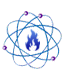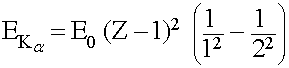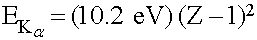Energy Dispersive X-ray Spectroscopy (EDAX)Scanning Electron Microscope with EDAX

Energy Dispersive X-ray Spectroscopy (abbreviated EDS, EDX, or EDAX after the company which manufactures a commonly used detector for the process) is an analysis tool used to determine the elemental composition of a sample. EDAX works by analyzing the spectrum of emitted X-rays from a sample as a beam of high energy electrons is incident upon its surface. By comparing the emitted X-ray photon energies to expected values from various elements one may determine which elements are present in a particular sample, and in what ratios.

During EDAX analysis, a beam of high energy electrons is directed at a sample which is to be studied. One of the high energy electrons incident upon the sample may act to excite an inner-shell electron out of one of the atoms making up the sample; in doing so a 'hole' is left in the inner shell where an electron should be when the atom is in a relaxed state. To return to the a relaxed state, one of the atom's outer-shell electrons quickly falls down into the hole left in the inner electron shell. In making this transition from a higher energy state (outer shell) to the lower energy state (inner shell), the electron emits a photon of energy which is detected as an X-ray.

The energy of the X-ray photon depends on the element of which the electron was a part, as well as the initial and final states of the electron making the transition. These energies are predictable.

X-ray producing transitions are commonly grouped into categories based on the initial and final states of the electron. These transitions are labeled as XJ, with X denoting the final state shell of the electron (K for n = 1, L for n = 2, M for n = 3, and so on), and J indicating how many shells above the final state the electron transitioned from (i = α, β, γ, … for 1, 2, 3, … respectively). For example, Lß would indicate that the electron transitioned to the n = 2 shell, from 2 shells above (n = 4).

Typically Kα (K-alpha) X-ray transitions are the strongest emission lines seen, which occur when an electron transitions from the n = 2 shell to the n = 1 shell. The energy given off (in the form of an X-ray photon) can calculated as,Where E0 = 13.6 eV, and Z (an integer ≥ 2) is the atomic number of the atom
This simplifies to,Further refinements can be made by taking into account more of the quantum numbers describing the states from which the electrons transition to / from, such as the angular momentum and spin, as well as selection rules describing which transitions are allowed.

An X-ray detector measures the number of photons of a particular energy given off by the sample. Over time, certain energy values will be found to occur much more frequently than others; these energy peaks correspond to the transitions of electrons around atoms of particular elements in the sample. By matching these energy peaks to the known / predicted values, one can determine what elements are present in a sample. Furthermore, the height of each peak is proportional to the abundance of its corresponding element, so one can also calculate the ratio between elements in a sample.

EDAX is not a perfect analysis tool however; it does have a few drawbacks. Very light elements are difficult, or even impossible in some cases, to measure due to the low-energy transitions which occur in the atoms (Hydrogen does not emit X-rays), and design limitations of the equipment (such as the material used for the ‘window’ in the detector). EDAX will only allow one to know the elemental composition (and proportions) of a sample, it will not give information as to how the atoms of these elements are bonded or arranged. Additionally, EDAX will not measure a sample uniformly through a given volume. Most of the signal EDAX measures will come from near the surface of the sample. This is due to the fact that the incident electrons, as well as the emitted X-rays, can only penetrate through the material certain distances before they are absorbed (thus they would not produce a detectable signal and that data is never collected). In order to increase the measurement depth one can increase the energy of the incident electrons to tens of keV; higher energies penetrate deeper into the sample. In doing so, one can extend to the depth one analyzes to several hundred nanometers to several microns below the surface, depending on the material being studied.

Last updated: 10/27/2008

This page is best viewed at a screen resolution greater than 800x600 using Internet Explorer with JavaScript enabled.

© 2005 - Amazing Rust .com International Chemical Supply    Disclaimer